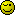I have created an array but the result show as one long number! How to separate them with a comma?

``````> \$query = \$db->simple_select("tags", "tid");

> while(\$theme = \$db->fetch_array(\$query))
> {
>     \$tids = array();

>     \$tids[] = \$theme['tid'];

>     \$hh = implode(', ', \$tids);
>   echo \$hh;
> }
``````

P.S. When I use print_r it show like this:
Array
(
 => 4
)
Array
(
 => 7
)
Array
(
 => 5
)
Array
(
 => 6
)
Array
(
 => 8
)

I’ll only ask a question, why is the above line in the loop, when it means you are resetting the variable back to an empty array with each iteration of the while loop? Oops, I said too much.Scott

I would like to fetch all tids from the TID column and then make them like this 1,2,3,4 etc. Then I will use this in the other query WHERE t.tid NOT IN

The implode should be outside of the while loop

It might be easier to just use a subquery in your WHERE statement too.

Scott

How can I fetch all values without the looping it?

Did you understand what Scott was saying in POST#2?

You define the array OUTSIDE the loop and then again implode would also be OUTSIDE the loop.

``````\$query = \$db->simple_select("tags", "tid");

\$tids = array();
while(\$theme = \$db->fetch_array(\$query))
{
\$tids[] = \$theme['tid'];
}
\$hh = implode(', ', \$tids);
echo \$hh;
``````

This topic was automatically closed 91 days after the last reply. New replies are no longer allowed.